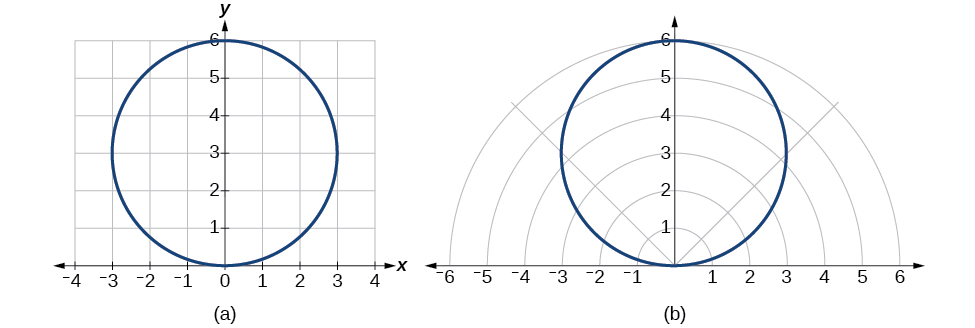10.3 Polar coordinates  (Page 3/8)

 Page 3 / 8

Writing a cartesian equation in polar form

Write the Cartesian equation $\text{\hspace{0.17em}}{x}^{2}+{y}^{2}=9\text{\hspace{0.17em}}$ in polar form.

The goal is to eliminate $\text{\hspace{0.17em}}x\text{\hspace{0.17em}}$ and $\text{\hspace{0.17em}}y\text{\hspace{0.17em}}$ from the equation and introduce $\text{\hspace{0.17em}}r\text{\hspace{0.17em}}$ and $\text{\hspace{0.17em}}\theta .\text{\hspace{0.17em}}$ Ideally, we would write the equation $\text{\hspace{0.17em}}r\text{\hspace{0.17em}}$ as a function of $\text{\hspace{0.17em}}\theta .\text{\hspace{0.17em}}$ To obtain the polar form, we will use the relationships between $\text{\hspace{0.17em}}\left(x,y\right)\text{\hspace{0.17em}}$ and $\text{\hspace{0.17em}}\left(r,\theta \right).\text{\hspace{0.17em}}$ Since $\text{\hspace{0.17em}}x=r\mathrm{cos}\text{\hspace{0.17em}}\theta \text{\hspace{0.17em}}$ and $\text{\hspace{0.17em}}y=r\mathrm{sin}\text{\hspace{0.17em}}\theta ,\text{\hspace{0.17em}}$ we can substitute and solve for $\text{\hspace{0.17em}}r.$

Thus, $\text{\hspace{0.17em}}{x}^{2}+{y}^{2}=9,r=3,$ and $\text{\hspace{0.17em}}r=-3\text{\hspace{0.17em}}$ should generate the same graph. See [link] .(a) Cartesian form   x 2 + y 2 = 9   (b) Polar form   r = 3

To graph a circle in rectangular form, we must first solve for $\text{\hspace{0.17em}}y.$

Note that this is two separate functions, since a circle fails the vertical line test. Therefore, we need to enter the positive and negative square roots into the calculator separately, as two equations in the form $\text{\hspace{0.17em}}{Y}_{1}=\sqrt{9-{x}^{2}}\text{\hspace{0.17em}}$ and $\text{\hspace{0.17em}}{Y}_{2}=-\sqrt{9-{x}^{2}}.\text{\hspace{0.17em}}$ Press GRAPH.

Rewriting a cartesian equation as a polar equation

Rewrite the Cartesian equation $\text{\hspace{0.17em}}{x}^{2}+{y}^{2}=6y\text{\hspace{0.17em}}$ as a polar equation.

This equation appears similar to the previous example, but it requires different steps to convert the equation.

We can still follow the same procedures we have already learned and make the following substitutions:

Therefore, the equations $\text{\hspace{0.17em}}{x}^{2}+{y}^{2}=6y\text{\hspace{0.17em}}$ and $\text{\hspace{0.17em}}r=6\mathrm{sin}\text{\hspace{0.17em}}\theta \text{\hspace{0.17em}}$ should give us the same graph. See [link] .(a) Cartesian form   x 2 + y 2 = 6 y (b) polar form   r = 6 sin   θ

The Cartesian or rectangular equation is plotted on the rectangular grid, and the polar equation is plotted on the polar grid. Clearly, the graphs are identical.

Rewriting a cartesian equation in polar form

Rewrite the Cartesian equation $\text{\hspace{0.17em}}y=3x+2\text{\hspace{0.17em}}$ as a polar equation.

We will use the relationships $\text{\hspace{0.17em}}x=r\mathrm{cos}\text{\hspace{0.17em}}\theta \text{\hspace{0.17em}}$ and $\text{\hspace{0.17em}}y=r\mathrm{sin}\text{\hspace{0.17em}}\theta .$

Rewrite the Cartesian equation $\text{\hspace{0.17em}}{y}^{2}=3-{x}^{2}\text{\hspace{0.17em}}$ in polar form.

$r=\sqrt{3}$

Identify and graph polar equations by converting to rectangular equations

We have learned how to convert rectangular coordinates to polar coordinates, and we have seen that the points are indeed the same. We have also transformed polar equations to rectangular equations and vice versa. Now we will demonstrate that their graphs, while drawn on different grids, are identical.

Graphing a polar equation by converting to a rectangular equation

Covert the polar equation $\text{\hspace{0.17em}}r=2\mathrm{sec}\text{\hspace{0.17em}}\theta \text{\hspace{0.17em}}$ to a rectangular equation, and draw its corresponding graph.

The conversion is

Notice that the equation $\text{\hspace{0.17em}}r=2\mathrm{sec}\text{\hspace{0.17em}}\theta \text{\hspace{0.17em}}$ drawn on the polar grid is clearly the same as the vertical line $\text{\hspace{0.17em}}x=2\text{\hspace{0.17em}}$ drawn on the rectangular grid (see [link] ). Just as $\text{\hspace{0.17em}}x=c\text{\hspace{0.17em}}$ is the standard form for a vertical line in rectangular form, $\text{\hspace{0.17em}}r=c\mathrm{sec}\text{\hspace{0.17em}}\theta \text{\hspace{0.17em}}$ is the standard form for a vertical line in polar form.

A similar discussion would demonstrate that the graph of the function $\text{\hspace{0.17em}}r=2\mathrm{csc}\text{\hspace{0.17em}}\theta \text{\hspace{0.17em}}$ will be the horizontal line $\text{\hspace{0.17em}}y=2.\text{\hspace{0.17em}}$ In fact, $\text{\hspace{0.17em}}r=c\mathrm{csc}\text{\hspace{0.17em}}\theta \text{\hspace{0.17em}}$ is the standard form for a horizontal line in polar form, corresponding to the rectangular form $\text{\hspace{0.17em}}y=c.$

A laser rangefinder is locked on a comet approaching Earth. The distance g(x), in kilometers, of the comet after x days, for x in the interval 0 to 30 days, is given by g(x)=250,000csc(π30x). Graph g(x) on the interval [0, 35]. Evaluate g(5)  and interpret the information. What is the minimum distance between the comet and Earth? When does this occur? To which constant in the equation does this correspond? Find and discuss the meaning of any vertical asymptotes.
The sequence is {1,-1,1-1.....} has
how can we solve this problem
Sin(A+B) = sinBcosA+cosBsinA
Prove it
Eseka
Eseka
hi
Joel
June needs 45 gallons of punch. 2 different coolers. Bigger cooler is 5 times as large as smaller cooler. How many gallons in each cooler?
7.5 and 37.5
Nando
find the sum of 28th term of the AP 3+10+17+---------
I think you should say "28 terms" instead of "28th term"
Vedant
the 28th term is 175
Nando
192
Kenneth
if sequence sn is a such that sn>0 for all n and lim sn=0than prove that lim (s1 s2............ sn) ke hole power n =n
write down the polynomial function with root 1/3,2,-3 with solution
if A and B are subspaces of V prove that (A+B)/B=A/(A-B)
write down the value of each of the following in surd form a)cos(-65°) b)sin(-180°)c)tan(225°)d)tan(135°)
Prove that (sinA/1-cosA - 1-cosA/sinA) (cosA/1-sinA - 1-sinA/cosA) = 4
what is the answer to dividing negative index
In a triangle ABC prove that. (b+c)cosA+(c+a)cosB+(a+b)cisC=a+b+c.
give me the waec 2019 questionsByByBy Lakeima RobertsBy Anindyo MukhopadhyayBy